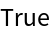Function Repository Resource:

# ProperLeafCount

Count the terminal nodes of an expression tree

 ResourceFunction["ProperLeafCount"][expr] counts terminal nodes of the tree graph associated with atomistic tree expression expr.

## Details

An atomistic tree expression is an expression that does not contain compound heads.

## Examples

### Basic Examples (1)

Count leaves of binary trees:

 In:=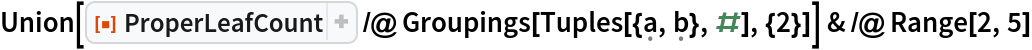Out=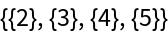### Properties and Relations (2)

ProperLeafCount is usually less than LeafCount:

 In:=Out=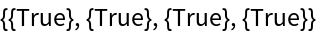In at least one trivial case, ProperLeafCount can equal LeafCount:

 In:=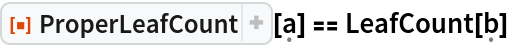Out=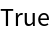### Possible Issues (1)

For nonatomistic inputs, a similar atomistic form is assumed:

 In:=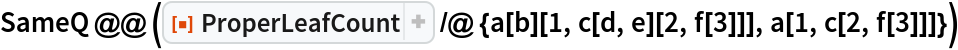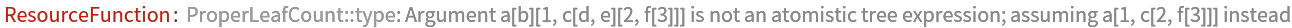Out=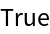### Neat Examples (1)

Compare ProperLeafCount with TreeCount:

 In:=Out=